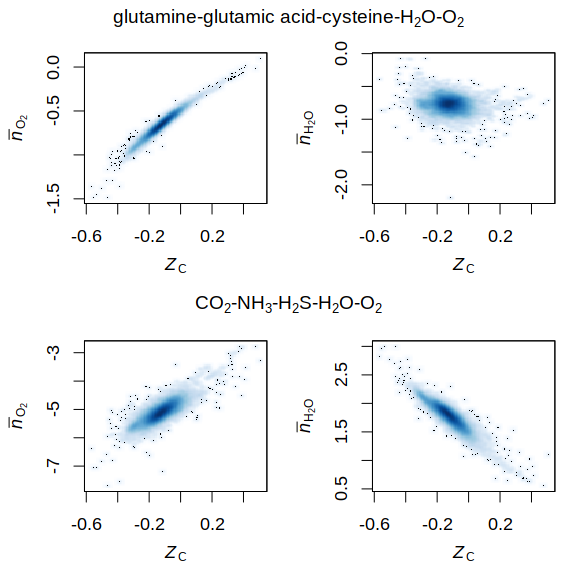# Basis Species Comparison

This vignette from the R package canprot shows projections of protein composition made using two sets of basis species. Using the CHNOS basis species (CO2, NH3, H2S, H2O, O2), the plots show that n̅H2O and n̅O2, i.e. the number of H2O and O2 in the formation per residue of the proteins from basis species, are both moderately correlated with ZC (average oxidation state of carbon). Using the QEC basis species (glutamine, glutamic acid, cysteine, H2O, O2), we find that n̅O2 is strongly correlated with ZC, but n̅H2O shows very little correlation. Accordingly, the QEC basis more clearly exposes two compositional variables – oxidation state and hydration state – in proteomic data.

First, load the canprot and CHNOSZ packages.

library(canprot)
library(CHNOSZ)

Here we define some labels used in the plot.

nH2Olab <- expression(bar(italic(n))[H * O])
nO2lab <- expression(bar(italic(n))[O])
ZClab <- expression(italic(Z)[C])
QEClab <- CHNOSZ::syslab(c("glutamine", "glutamic acid", "cysteine", "H2O", "O2"))
CHNOSlab <- CHNOSZ::syslab(c("CO2", "NH3", "H2S", "H2O", "O2"))

Next, get the amino acid compositions of all proteins in the UniProt human proteome and calculate the protein formulas and ZC. Note that ZC is a sum of elemental ratios and is independent of the choice of basis species.

aa <- get("human_base", canprot)
protein.formula <- CHNOSZ::protein.formula(aa)
ZC <- CHNOSZ::ZC(protein.formula)

Now set up the figure and plot the per-residue compositions of the proteins projected into different sets of basis species.

par(mfrow = c(2, 2))
par(mar = c(4, 4, 2.5, 1))
par(cex = 1.1)
par(mgp = c(2.5, 1, 0))
for(basis in c("QEC", "CHNOS")) {
CHNOSZ::basis(basis)
protein.basis <- CHNOSZ::protein.basis(aa)
protein.length <- CHNOSZ::protein.length(aa)
residue.basis <- protein.basis / protein.length
smoothScatter(ZC, residue.basis[, "O2"], xlab = ZClab, ylab = nO2lab)
smoothScatter(ZC, residue.basis[, "H2O"], xlab = ZClab, ylab = nH2Olab)
if(basis=="QEC") mtext(QEClab, outer = TRUE, cex = 1.2, line = -1.5)
if(basis=="CHNOS") mtext(CHNOSlab, outer = TRUE, cex = 1.2, line = -15)
}This figure has been published as Figure S1 of Dick, 2017 (Chemical composition and the potential for proteomic transformation in cancer, hypoxia, and hyperosmotic stress. PeerJ 5:e3421).